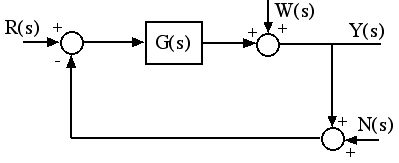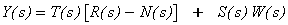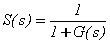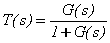Consider the unity-feedback control system:where
• r(t) is the reference input signal,
• w(t) is a disturbance input signal, typically a low frequency signal,
• n(t) is a measurement (sensor) noise signal, typically a high frequency signal,
• y(t) is the output signal,
• G(s) is a strictly-proper rational transfer function representing the plant and compensator.

We view this as a tracking system, where the objective is that the output signal track the reference input signal and reject the influences of the disturbance and noise input signals.

In terms of Laplace transforms, the control system response is described bywhere the transfer functions in this expression are

Sensitivity Function:Complementary Sensitivity Function:The ideal objective (perfect tracking) is to have Y(s) = R(s), regardless of N(s) and W(s). Usually this is interpreted in terms of frequency response functions, where the ideal objective is Y(jw ) = R(jw ), for all w. Of course, the ideal objective is unattainable, and furthermore, for simplicity, we often interpret the objective in terms of magnitudes only. That is, we would like to have |Y(jw )| = |R(jw )|, for all w.

The typical situation is that |R(jw )|, |W(jw )| are small for large w , and |N(jw )| is small for small w .

Thus for good tracking performance, we require that

• |S(jw )| be small for small w , so that the effect of the disturbance input is attenuated,
• |T(jw )| be small for large w , so that the effect of the sensor noise is attenuated,
• |T(jw )| be unity ( 0 db) for small w so that the (low-frequency) characteristics of the reference input are unaffected.

These requirements for good tracking performance also can be considered in terms of characteristics of a typical plant/compensator transfer function G(s). If |G(jw )| is large for small w , then |T(jw )| will be approximately unity and |S(jw )| will be large for small w . If |G(jw )| is small for large w , then |T(jw )| will be small and |S(jw )| will be approximately unity for large w . For such a G(s), additional characteristics of tracking performance depend on the behavior of both |G(jw )| and Ð G(jw ) in the mid-range of frequency, particularly where |G(jw )| is near 0 db and Ð G(jw ) is near -180 deg.

These issues can be explored using the applet below. The plant/compensator transfer function has the formwhere k, a, b and c are positive, real coefficients that can be adjusted by dragging the marked corner frequencies, a, b, and c, on the Bode magnitude plot for G(s). (The "pole" corner frequencies are marked with x's.) Also marked are the gain crossover frequency and the phase crossover frequency. The corresponding Bode magnitude plots for T(s) and S(s) are shown. Magnitude plots are in units of decibels, and phase plots are in degrees. Also there are windows where a disturbance signal w(t) can be drawn with the mouse, the sensor noise signal n(t) can be selected, the reference input signal r(t) can be drawn, and the corresponding output signal y(t) is displayed. Note that a convenient way to zero a sketched signal is to sketch a short horizontal line at zero. Numerical values of the plant coefficients, gain and phase crossover frequencies, and gain and phase margins are shown. The Integral Square Error between the reference input and the output is computed on the range 0 < t < 15.

The initial G(s) in the applet is such that the closed-loop system is unstable. Therefore the first task is to drag corner frequencies to stabilize the system. Also the "zero" corner frequency, c, initially is given a large value so that it has relatively little effect on the system behavior. It is simpler to explore this case first. When you wish to explore the effect of the zero, note that the zero corner frequency cannot be dragged too close to a pole corner frequency.return to demonstrations page

 Applet by Seth Kahn